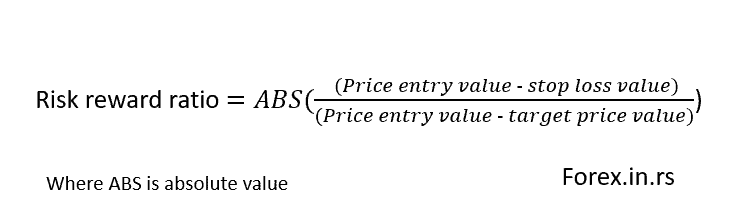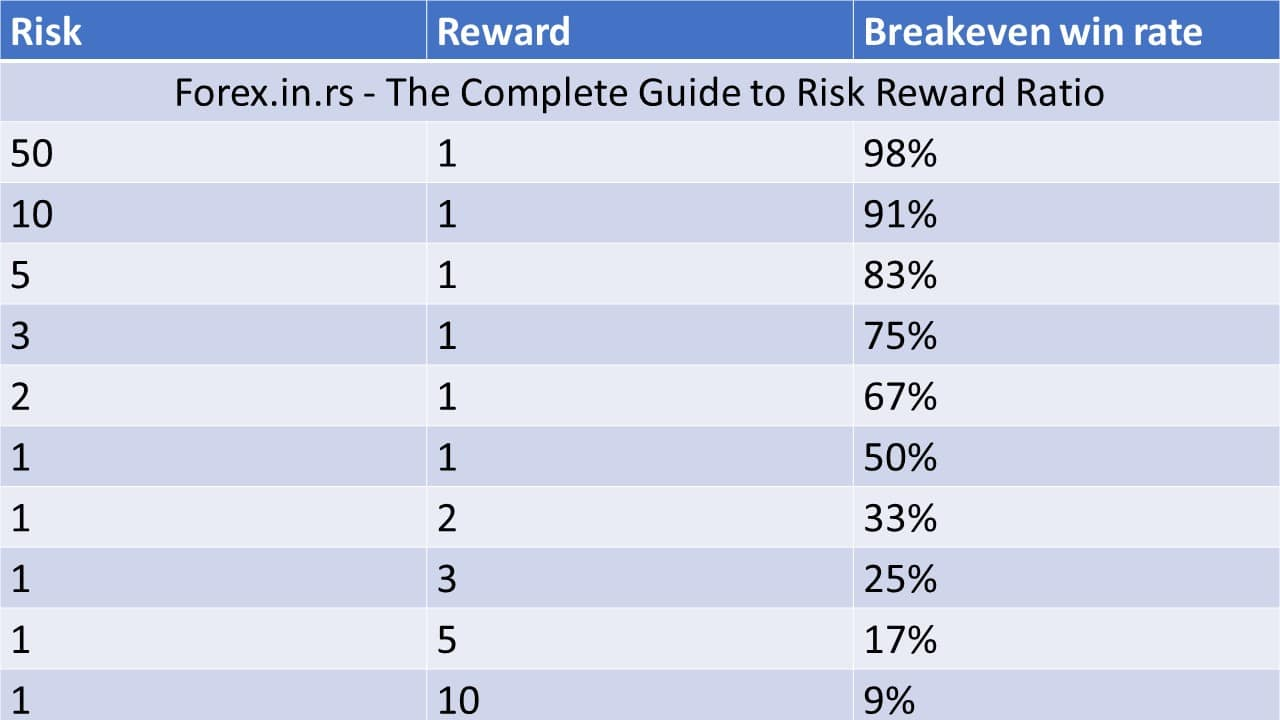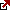# Risk Return Ratio – Risk Reward Ratio Explained

The risk vs. reward in investing represents the prospective reward an investor can earn from an investment for every dollar that investor risks on an investment.

But in trading, the risk-reward ratio in trading is slightly different and has a different weight of significance.

Whether you are an experienced trader or a new one, the risk-reward ratio can be a tricky thing to understand. You might feel that you are in complete control, and the set ratio will always work for you, but such is seldom the case. To take the maximum advantage of this ratio, you must pay attention to the details.

What is the Risk-Reward Ratio?
The risk-reward ratio or risk-return ratio in trading represents the prospective reward an investor can earn from an investment for every dollar that investor risks on investment. The risk-return ratio can be calculated as the expected return and risk of a given trade or trades based on entry position and close position. A good risk-reward ratio tends to be less than 1; that is, the return (reward) is greater than the risk.

Risk-reward ratio formula (risk-return ratio formula):Trading is nerve linear. No matter how stable the market looks, there is always a certain degree of risk involved. The risk-reward ratio is an estimation of a possible reward for every dollar that you trade. It shows the proportion of your expenditure and potential reward.

For example, consider that you are operating with a 1:4 risk-reward ratio. Ideally, it would mean that for every dollar that you spend, you will get a reward of \$4. Similarly, if the ratio is 1:6, you will get \$6 for every \$1 spent. The higher the ratio, the more will be the reward, but that doesn’t always work. As a single metric system, the risk-reward ratio holds no meaning.

The risk-reward forex ratio tells you about your possible profit against your investment. Your capital or investment is the ‘risk,’ and your target profit is the ‘reward.’ Here’s an example:

Consider placing a trade, and your stop loss is set at 5 pips, and your take profit is set at 20 pips. In this case, the ratio is 5:20. The simple conversion would be 1:4. You expect to make a profit of \$4 on every dollar you spend in terms of money. Or you have a chance of winning 20 pips by risking 5 pips.

So the risk-reward ratio in investing and risk-reward ratio in trading has a different weight of significance. Investing in the risk-return ratio (the risk-reward ratio) is very important, and if investors can invest \$1 and earn \$5 (1/5 risk-reward ratio) will be very profitable. But in trading high risk-reward ratio has a low impact on trading performance without a winning rate.

For example, if the trader has \$1 risk and  has a 1/5 risk-reward and only a 10% winning rate, then after 100 trades, he will get:

Summary Loss: \$40

So, traders need to analyze the winning rate and risk-reward ratio to make the best conclusion. See the minimum winning rate for each risk-reward ratio below:Free Risk-reward calculator:

The Ideal Risk-Reward Ratio

Many brokers and veteran traders will suggest that you must not accept less than the 1:2 risk-reward ratio. It might work in your favor, and it might not. To give a concrete answer without studying it alongside other variables would be a futile act. Let’s see how.

Let’s assume that your risk-reward ratio is 1:2, where you will gain \$2 for every \$1 investment. With this ratio, you place 20 trades but only win 4. Your winning percentage is 20% only. The following calculation will show you how much you will actually win:

Total Losses: 16 * \$1 = -\$16

Total Profit: 4 * \$2 = \$8

Net Loss: \$8

This proves that the risk-reward ratio doesn’t tell you anything unless combined with the winning rate.

How to Calculate Expectancy instead of the Risk-Reward Ratio for Your Benefit

The risk-reward ratio is applicable in every trade of every security, but you can make the most out of it only when you know how to calculate the risk-reward ratio in Forex, stocks, futures, and more. Forget about the old formulas and use the one given below:

E = (W/L) * P – 1

Confused? Let us explain.

In the above formula, ‘W’ is the size of your average win, ‘L’ denotes average loss, and ‘P’ stands for the winning rate.

Now let’s use this formula to see your actual reward.

Let’s assume that you are making 10 trades, out of which you lost 4 and won 6. Your winning percentage is 60%. Suppose that these wins gained you \$3000, and you gained \$500 on an average. Let us also assume that you lost \$1600 against the 4 losses (average loss of \$400). Let’s put the values in the formula:

E= [1+ (500/400)] x 0.6 – 1 = 0.35 or 35%.

Here, your ‘E’ or expectancy is positive (35%). This means that if we consider long-term trade, your gain will be 35 cents on every dollar spent.

Using Tools to Define the Risk

Some tools can help traders in managing their losses. Stop-loss is one such tool. You can use it as a barrier to keep the prices from going beyond the prices you have set. It is like a market structure that saves you. This is how you can use it:

1. Support Resistance

Support is the area that is susceptible to buying pressure, and Resistance is the area that can feel the selling pressure. You can take profits at Resistance in a long position, and if you are in a short position, profits can be taken at Support. You can take advantage of this technique when the market is in a week trend or a range.

While using this technique, don’t aim for absolute highs and lows because there are chances that the market may never reach that.

2. Fibonacci Extension

You can project the current swing extension using the Fibonacci extension. The possible extensions are 127, 132, and 162. During a weak or healthy trend, the prices can go beyond the previous swing high and retrace to a lower level, known as the uptrend; the extension is useful.

The Fibonacci extension allows you to predict how high the prices will reach and your exit point before the prices retrace.

The Fibonacci extension is easy to use. First, you need to identify a trending market and then draw a Fibonacci extension tool. Do it from high swing to low swing. Set a target using any of the three extensions stated above. Do the opposite in an uptrend.

3. Chart Pattern Completion

The markets tend to find exhaustion on the completion of a chat pattern. This is a classical principle of charting. The completion of the chart refers to the price movement of an equal distance.

Analyzing the Risk-Reward Ratio

Once you understand how a stop loss works and set a target profit, it’s time to again calculate the risk-reward ratio by teaming it up with stop loss. This can be done in three steps:

• What is the stop-loss distance
• What is the target profit distance
• The difference between the above two

Here is an example for your reference:

Let’s consider that your stop loss is 100 pips while the target profit is 200 pips. Your risk-reward ratio would be:

200/100 = 2

Ratio = 1:2

The risk-reward ratio becomes important when the prices are near resistance and support. Let’s assume that the currency pair JPY/USD is in a downtrend. If the prices have paused near resistance, the risk-reward ratio will most probably favor a sell trade as the risk is on the lower side. You can put a smaller stop a little above the entry and a comparatively larger profit along the trend’s direction.

The Risk-Reward Ratio is Not Everything

We have proved it with enough examples that the risk-reward ratio cannot help you alone, and you need to use it along with your winning rate. However, if you are still losing money, many other variables can help you make a difference. Trend Trading is one such variable. It is a big part of the trading market. Your chances of making profits will certainly increase if you use trends. Both new and experienced traders can use this technique.

A stop-loss is another beneficial tool that can help you in risk-management.

The Kinds of Risk-Reward Ratio that Traders Must Consider

One bill doesn’t fit all, and the same goes with the risk-reward ratio. The risk-reward type that will suit you the most will depend on the market conditions and your trading style. A general assumption is that if your reward is high and the risk is low, you will remain in a profitable situation. While this does sound ideal, it is not always realistic.

Traders do not accept less than a 1:2 risk-reward ratio, and it does seem plausible. Why would you risk more than your expected reward? However, the market is volatile; it might work for you. You can use the stop loss to protect your investment and to alert you when the trade no longer seems profitable. Taking more risks should only be practiced by experienced traders and investors.

Conclusion

The risk-reward ratio type varies greatly. It could be 1:2, 1:5, 1:6, and even 5:1. Your experience and your trading style will determine which type of ratio is best suited to you. Ideally, rewards should exceed the risk, but you can evaluate the market and go the other way if you are experienced and confident. As a solitary measure, the risk-reward ratio cannot define your path to success. You must see your winning expectancy, the condition of the market, your trading style, and the risk that you can afford to take without creating a financial burden for yourself.

There is no magical formula, tool, or feature that can make trading absolutely profitable or safe. The risk-reward ratio is one of the many variables that can help you make better trading strategies that can attract higher profit. Risk-reward is a good tool and can be a game-changer when used with other aspects.

Latest posts by Fxigor (see all)

Fxigor

Igor has been a trader since 2007. Currently, Igor works for several prop trading companies. He is an expert in financial niche, long-term trading, and weekly technical levels. The primary field of Igor's research is the application of machine learning in algorithmic trading. Education: Computer Engineering and Ph.D. in machine learning. Igor regularly publishes trading-related videos on the Fxigor Youtube channel. To contact Igor write on: igor@forex.in.rs

Trade gold and silver. Visit the broker's page and start trading high liquidity spot metals - the most traded instruments in the world.Diversify your savings with a gold IRA.

Gold & silver portfolio-building assistance from product professionals.

If you want to trade stocks try: